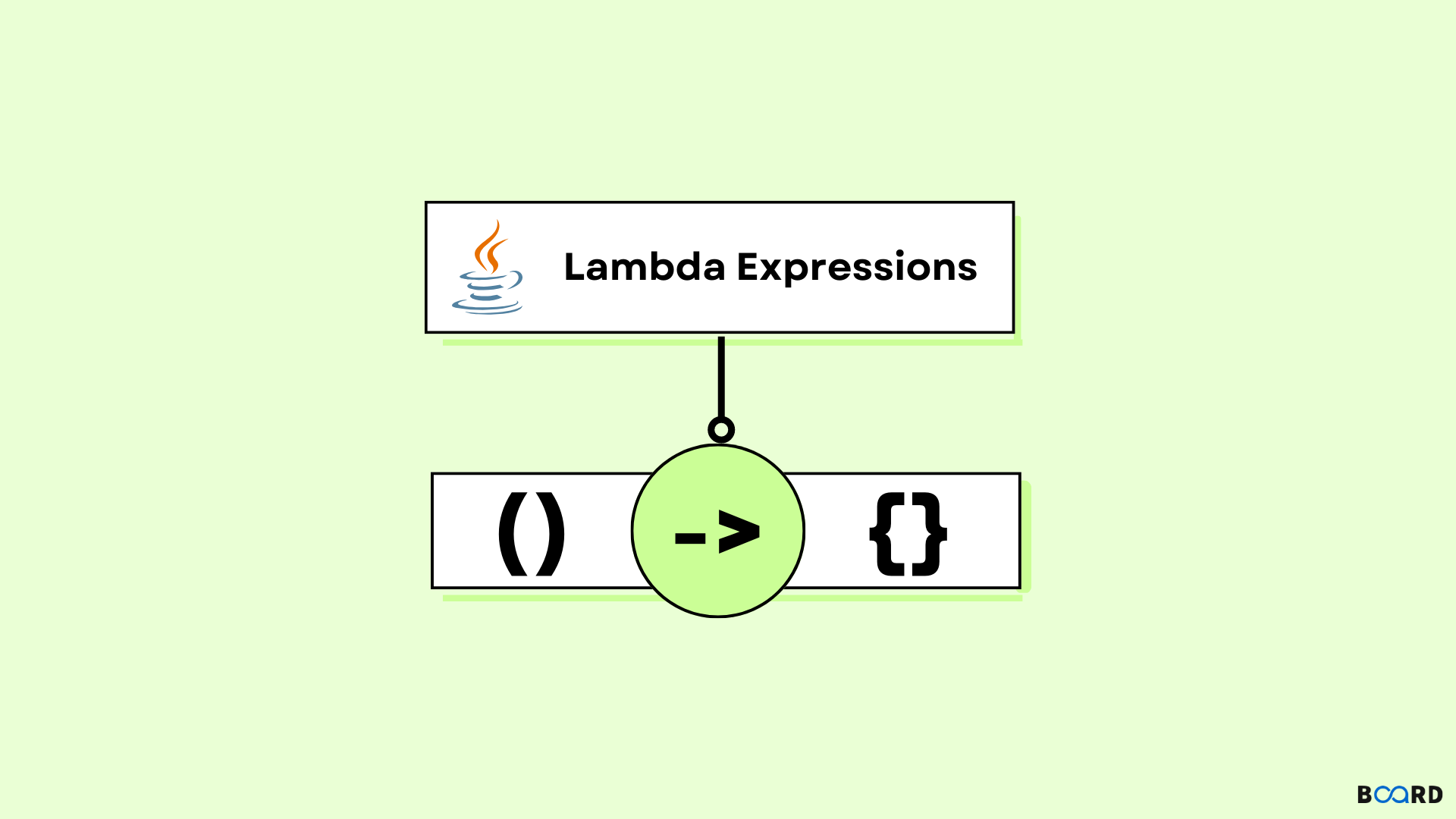# Lambda Expression in Java## Introduction

In Java SE 8, an important and new feature was introduced Lambda Expressions. Using an expression provides a clear and brief way to express method interfaces. It does help in the collection library as it helps in iterating, filtering and extracting data from a collection.

The compiler doesn’t create a .class file because lambda expression is handled as a function.

## Functional Interface

The Functional interface is an interface that only has one abstract method. To declare a functional interface, java provided an annotation i.e. @FunctionalInterface. Lambda expressions give the implementation of a functional interface.

## Benefits of Lambda Expression

• Handles the functionality as a method argument or code as data. It saves a lot of code. We do not need to define the method again, for the implementation part, here we just need to type out the implementation code.
• When needed lambda expression can be used as an object and can also be passed around.
• Not being in any class, a function can be created.
• Also gives the implementation of a functional interface.

## Java Lambda Expression Syntax

 (argument-list) -> {body}

Java lambda expression has three components.

1. Argument list: It is empty and also can be non-empty.
2. Arrow-token: The arrow token is used to link the body expressions with the arguments list.
3. Body: It has the statements for the lambda expressions.

### No parameter syntax

 () -> {  //Body}

### One Parameter Syntax

 (p1) -> {//Body}

### Two Parameter Syntax

 (p1,p2) -> { //Body}

## No Parameter Java Lambda Expression

Code

 interface Interface{     public String print();  }  public class main{    public static void main(String[] args) {      Interface s=()->{          return "Hello User";      };      System.out.println(s.print());    }  }

Output

 Hello User
write your code here: Coding Playground

## Single Parameter Java Lambda Expression

Code

 interface Interface{      public String print(String name);  }    public class main{      public static void main(String[] args) {                // Single parameter.          Interface I1=(name)->{              return "Hey, "+name;          };          System.out.println(I1.print("User"));                    // Function parentheses omitted        Interface I2= name ->{              return "Hey, "+name;          };          System.out.println(I2.say("User"));    }  }

Output

 Hey UserHey User
write your code here: Coding Playground

## Multiple Parameters Java Lambda Expression

Code

 interface add{      int calc(int x,int y);  }    public class main{      public static void main(String[] args) {                    // Multiple parameters          add a1=(x,y)->(x+y);          System.out.println(a1.calc(10,20));                    // Multiple parameters        add a2=(int x,int y)->(x+y);          System.out.println(a2.calc(100,200));      }  }

Output

 30300
write your code here: Coding Playground

## For each loop using Java Lambda Expression

Code

 import java.util.*;  public class main{      public static void main(String[] args) {                    List l=new ArrayList();          list.add("l1");          list.add("l2");          list.add("l3");          list.add("l4");                    list.forEach(              (x)->System.out.println(x)          );      }  }

Output

 l1l2l3l4
write your code here: Coding Playground

## Multiple Statements Java Lambda Expression

Code

 @FunctionalInterface  interface Interface{      String print(String s);  }    public class main{      public static void main(String[] args) {                // Passing multiple statements          Interface I = (s)-> {              String s1 = "Hello ";              String s2 = str1 + message;               return s2;          };              System.out.println(I.print("World! "));      }  }

Output

 Hello World!
write your code here: Coding Playground